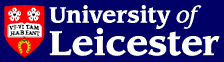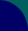# School of Mathematics & Computer Science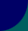# Technical Reports 2003

## Summary

2003/01 Michelle Vail.

Error Estimates for Spaces Arising from Approximation by Translates of a Basic Function (PhD Thesis)

2003/02 Paola Frediani and Frank Neumann.

Etale Homotopy Types of Moduli Stacks of Algebraic Curves with Symmetries

2003/03 John Hunton and Mikhail Shchukin.

The $K$-theory of $C^*$-algebras with finite dimensional irreducible representations

2003/04 Frank Neumann and Ulrich Stuhler.

Moduli Stacks of Vector Bundles and Frobenius Morphisms

2003/05 Phillipe Caldero and Robert Marsh.

A multiplicative property of quantum flag minors II

2003/06 Jeremy Levesley.

Perturbed kernel approximation on homogeneous manifolds

2003/07 Volodymyr Mazorchuk and Catharina Stroppel.

Translation and shuffling of projectively presentable modules and a categorification of a parabolic Hecke module

2003/08 Teijo Arponen.

2-Tensor invariants in numerical integration

2003/10 Claire Irving.

Embeddings and Immersions of Real Projective Spaces

2003/12 R. J. Marsh and K. Rietsch.

Parametrizations of flag varieties.

2003/13 Steffen Koenig.

Filtrations, Stratifications and Applications

2003/14 P. Houston, I. Perugia and D. Schotzau.

Discontinuous Galerkin Methods for Maxwell's Equations

2003/15 P. Houston, I. Perugia and D. Schotzau.

Nonconforming Mixed Finite Element Approximations to Time-Harmonic Eddy Current Problems

2003/16 P. Houston, I. Perugia and D. Schotzau.

Energy Norm A Posteriori Error Estimation for Mixed Discontinuous Galerkin Approximations of the Maxwell Operator

2003/17 P. Houston, I. Perugia and D. Schotzau.

Mixed Discontinuous Galerkin Approximation of the Maxwell Operator: Non-stabilized Formulation

2003/18 P. Houston, D. Schotzau and T. P. Wihler.

Energy Norm A Posteriori Error Estimation for Mixed Discontinuous Galerkin Approximations of the Stokes Problem

2003/19 J. Levesley.

Where there's a Will there's a Way -- the Research of Will Light

2003/20 Gerhard Rosenberger, Martin Scheer and Richard M. Thomas.

Finite generalized tetrahedron groups with a cubic relator

2003/21 Vincent Schmitt.

Flatness, preorders and general metric spaces.

2003/22 M. Felisatti.

Multiplicative K-theory and K-theory of functors

2003/23 Steffen Koenig, Oleksandr Khomenko and Volodymyr Mazorchuk.

Finitistic dimension and tilting modules for stratified algebras

2003/24 Rick Thomas.

A course on permutation groups

2003/26 Karin Erdmann, Miles Holloway, Nicole Snashall, Oeyvind Solberg and Rachel Taillefer.

Support Varieties for Selfinjective Algebras

2003/27 A. A. Baranov.

Classification of direct limits of involution simple associative algebras

2003/28 Y.A. Bahturin, A.A. Baranov and A.E. Zalesskii.

Simple Lie subalgebras of locally finite associative algebras

2003/29 A.A. Baranov and I.D. Suprunenko.

Modular branching rules for 2-column diagram representations of general linear groups

## Full details

2003/01 Michelle Vail.

Error Estimates for Spaces Arising from Approximation by Translates of a Basic Function (PhD Thesis)

We look at aspects of error analysis for interpolation by translates of a basic function. In particular, we consider ideas of localisation and how they can be used to obtain improved error estimates. We shall consider certain seminorms and associated spaces of functions which arise in the study of such interpolation methods. These seminorms are naturally given in an indirect form, that is in terms of the Fourier Transform of the function rather than the function itself. Thus, they do not lend themselves to localisation. However, work by Levesley and Light rewrites these seminorms in a direct form and thus gives a natural way of defining a local seminorm. Using this form of local seminorm we construct associated local spaces. We develop bounded, linear extension operators for these spaces and demonstrate how such extension operators can be used in developing improved error estimates. Specifically, we obtain improved $L_2$ estimates for these spaces in terms of the spacing of the interpolation points. Finally, we begin a discussion of how this approach to localisation compares with alternatives.

2003/02 Paola Frediani and Frank Neumann.

Etale Homotopy Types of Moduli Stacks of Algebraic Curves with Symmetries

Using the machinery of etale homotopy theory \`{a} la Artin-Mazur we determine the etale homotopy types of moduli stacks over $\bar{\Q}$ parametrizing families of algebraic curves of genus $g \geq 2$ endowed with an action of a finite group $G$ of automorphisms, which comes with a fixed embedding in the mapping class group $\Gamma_g$, such that in the associated complex analytic situation the action of $G$ is precisely the differentiable action induced by this specified embedding of $G$ in $\Gamma_g$

2003/03 John Hunton and Mikhail Shchukin.

The $K$-theory of $C^*$-algebras with finite dimensional irreducible representations

We study the $K$-theory of unital $C^*$-algebras $A$ satisfying the condition that all irreducible representations are finite and of dimension at most some integer $n$. When the algebra $A$ is $n$-homogeneous, {\em i.e.}, all irreducible representations are exactly of dimension $n$, we show $K_*(A)$ is the topological $K$-theory of a related compact Hausdorff space, generalising the classical Gelfand-Naimark theorem. For general $A$ we give a spectral sequence computing $K_*(A)$ from a sequence of topological $K$-theories of related spaces. For $A$ generated by two idempotents, this becomes a 6-term long exact sequence.

2003/04 Frank Neumann and Ulrich Stuhler.

Moduli Stacks of Vector Bundles and Frobenius Morphisms

We describe the action of the different Frobenius morphisms on the etale cohomology ring of the moduli stack of algebraic vector bundles of fixed rank and determinant on an algebraic curve over a finite field in characteristic p and analyse special situations like vector bundles on the projective line and relations with infinite Grassmannians.

2003/05 Phillipe Caldero and Robert Marsh.

A multiplicative property of quantum flag minors II

Let U^+ be the plus part of the quantized enveloping algebra of a simple Lie algebra of type A_n and let B^* be the dual canonical basis of U^+. Let b, b' be in B^* and suppose that one of the two elements is a q-commuting product of quantum flag minors. We show that b and b' are multiplicative if and only if they q-commute.

Available as: gzipped PostScript (.ps.gz)

2003/06 Jeremy Levesley.

Perturbed kernel approximation on homogeneous manifolds

Current methods for proving the existence of unique interpolants for kernel approximation require the kernels to be conditionally positive definite. The subsequent convergence rates for interpolants require the same condition. In this paper we show how this requirement may be relaxed, if the kernel being used to approximate with is a smooth perturbation of a positive definite kernel.

2003/07 Volodymyr Mazorchuk and Catharina Stroppel.

Translation and shuffling of projectively presentable modules and a categorification of a parabolic Hecke module

We investigate the category of $\p$-presentable modules in the principal block of the Bernstein-Gelfand-Gelfand category $\Oo$. This category is equivalent to the module category of a properly stratified algebra. We describe the socles and endomorphism rings of standard objects in this category. We consider translation and shuffling functors and their action on the standard modules. Finally, we study a graded version of this category, in particular, giving a graded version of the properly stratified structure, and using graded versions of translation functors to categorify a parabolic Hecke module.

2003/08 Teijo Arponen.

2-Tensor invariants in numerical integration

In geometric integration of ordinary differential equations (ODEs) one needs concepts which are both geometric and algebraic. In this paper we start from the algebraic point of view: we introduce the 2-tensor invariants attached to an ODE. These generalize the well known notion of a symplectic structure.

2003/10 Claire Irving.

Embeddings and Immersions of Real Projective Spaces

In this document, rigorous definitions of the concepts of immersions and embeddings of manifolds are set out. Whitney's immersion and embedding theorems are proved and stronger versions of the theorems are quoted. The real projective plane is considered in detail; an immersion and an embedding of $\mathbb{R}P^{2}$ are given, along with the results that $\mathbb{R}P^{2}$ cannot be immersed into $\mathbb{r}^{2}$ and cannot be embedded into $\mathbb{R}^{3}$. A non-immersion theorem for $\mathbb{R}P^{2^{r}}$ is proved using Stiefel-Whitney classes and a non-embedding result for $\mathbb{R}P^{n}$, where $n$ is not a power of 2, is proved using cohomology and cohomology operations. Finally, $K$-theory is introduced and used to prove two theorems, one giving dimensions of Euclidean space into which $\mathbb{R}P^{n}$ cannot be embedded or immersed and the other giving possible immersions for $\mathbb{R}P^{n}$.

2003/12 R. J. Marsh and K. Rietsch.

Parametrizations of flag varieties.

For the flag variety G/B of a reductive algebraic group G we define a certain (set-theoretical) cross-section phi from G/B to G, which depends on a choice of reduced expression for the longest element in the Weyl group. This cross-section is continuous along the components of Deodhar's decomposition of G/B and assigns to any flag gB a representative phi(gB) in G which comes with a natural factorization into simple root subgroups and simple reflections. We introduce a generalization of the Chamber Ansatz of Berenstein, Fomin and Zelevinsky and use it to obtain formulas for the factors of phi(gB). Our results then allow us parameterize explicitly the components of the totally nonnegative part of the flag variety as defined by Lusztig. This gives a new proof of Lusztig's conjectured cell decomposition of this set.

Available as: gzipped PostScript (.ps.gz)

2003/13 Steffen Koenig.

Filtrations, Stratifications and Applications

These are lecture notes from a course given at ICRA X in Toronto in 2002. Filtrations and stratifications define classes of algebras such as quasi-hereditary, cellular and stratified algebras. The use of their properties is illustrated by a number of examples, including Schur-Weyl duality, Soergel's structure theorem of the Bernstein-Gelfand- Gelfand category, Enright's conjecture, and identities of decomposition numbers.

2003/14 P. Houston, I. Perugia and D. Schotzau.

Discontinuous Galerkin Methods for Maxwell's Equations

We present discontinuous Galerkin methods for the numerical discretization of time-harmonic eddy current problems in conducting and insulating materials. The involved operators are discretized by using suitable variants of the classical interior penalty technique; here, the divergence-free constraint on the electric field in insulating materials is imposed either by using a regularization approach or by a Lagrange multiplier technique.

Available as: gzipped PostScript (.ps.gz)

2003/15 P. Houston, I. Perugia and D. Schotzau.

Nonconforming Mixed Finite Element Approximations to Time-Harmonic Eddy Current Problems

We present nonconforming mixed finite element methods for the discretization of time-harmonic eddy current problems. These methods are based on a discontinuous Galerkin approach, where the unknowns are approximated by completely discontinuous piecewise polynomials. In particular, we consider a stabilized mixed formulation involving equal-order elements, and a non-stabilized variant employing mixed-order elements.

Available as: gzipped PostScript (.ps.gz)

2003/16 P. Houston, I. Perugia and D. Schotzau.

Energy Norm A Posteriori Error Estimation for Mixed Discontinuous Galerkin Approximations of the Maxwell Operator

In this paper we develop the {\em a posteriori} error estimation of mixed discontinuous Galerkin finite element approximations of the Maxwell operator. In particular, by employing suitable Helmholtz decompositions of the error, together with the conservation properties of the underlying method, computable upper bounds on the error, measured in terms of a natural (mesh-dependent) energy norm, are derived. Numerical experiments testing the performance of our {\em a posteriori} error bounds for problems with both smooth and singular analytical solutions are presented.

Available as: gzipped PostScript (.ps.gz)

2003/17 P. Houston, I. Perugia and D. Schotzau.

Mixed Discontinuous Galerkin Approximation of the Maxwell Operator: Non-stabilized Formulation

A non-stabilized mixed discontinuous Galerkin method for the discretization of the time-harmonic Maxwell operator on simplicial meshes is studied. In contrast to the stabilized scheme introduced in , the proposed formulation contains no normal--jump stabilization; instead, it is based on discontinuous mixed-order elements for the approximation of the unknowns. Optimal a priori error bounds in the energy norm are derived; the error analysis relies on suitable decompositions of discontinuous spaces and on stability properties of the underlying conforming spaces. The formulation is tested on a set of numerical examples in two space dimensions.

Available as: gzipped PostScript (.ps.gz)

2003/18 P. Houston, D. Schotzau and T. P. Wihler.

Energy Norm A Posteriori Error Estimation for Mixed Discontinuous Galerkin Approximations of the Stokes Problem

In this paper, we develop the a posteriori error estimation of mixed discontinuous Galerkin finite element approximations of the Stokes problem. In particular, computable upper bounds on the error, measured in terms of a natural (mesh--dependent) energy norm, are derived. The proof of the a posteriori error bound is based on rewriting the underlying method in a non-consistent form by introducing appropriate lifting operators, and employing a decomposition result for the discontinuous spaces. A series of numerical experiments highlighting the performance of the proposed a posteriori error estimator on adaptively refined meshes are presented.

Available as: gzipped PostScript (.ps.gz)

2003/19 J. Levesley.

Where there's a Will there's a Way -- the Research of Will Light

This paper describes the work of Will Light, who died on December 8th, 2002. It highlights his contributions in the study of minimal norm projections, tensor product approximation (including the convergence of the Diliberto-Straus algorithm), proximality, radial and ridge function approximation, both via quasi-interpolation and interpolation. My aim is not only to describe the impact of Will's work, but also to convey some of his impact as a person.

2003/20 Gerhard Rosenberger, Martin Scheer and Richard M. Thomas.

Finite generalized tetrahedron groups with a cubic relator

An ordinary tetrahedron group is a group with a presentation of the form % $\langle x,y,z \mid x^{e_1}=y^{e_2}=z^{e_3}=(xy^{-1})^{f_1}=(yz^{-1})^{f_2}=(zx^{- 1})^{f_3}=1 \rangle$ % where $e_i\ge2$ and $f_i\ge2$ for all $i$. Following Vinberg, we call groups defined by a presentation of the form % $\langle x,y,z \mid x^{e_1}=y^{e_2}=z^{e_3}=R_1(x,y)^{f_1}=R_2(y,z)^{f_2}=R_3(z,x)^{f_3}=1 \rangle,$ % where each $R_i(a,b)$ is a cyclically reduced word involving both $a$ and $b$, generalized tetrahedron groups. These groups appear in many contexts, not least as subgroups of generalized triangle groups. In this paper, we build on previous work of Coxeter, Edjvet, Fine, Howie, Levin, Metaftsis, Roehl, Rosenberger, Stille, Thomas, Tsaranov and Vinberg (amongst others) to give a classification of the finite generalized tetrahedron groups with a cubic relator.

2003/21 Vincent Schmitt.

Flatness, preorders and general metric spaces.

This paper studies a general notion of flatness in the enriched context: p-flatness where the parameter p stands for a class of presheaves. One obtains a completion of a category A by considering the category Fp(A) of p-flat presheaves over A. This completion is related to the free-cocompletion under a class of colimits defined by Kelly. For a category A, for p = pz the class of all presheaves, Fpz(A) is the Cauchy-completion of A. Two classes Pu and Pd of interest for general metric spaces are considered. The pu and pd flatness are investigated and the associated completions are characterized for general metric spaces (enrichments over R) and preorders (enrichments over B). We get this way two non-symmetric completions for metric spaces and retrieve the ideal completion for preorders.

Available as: gzipped PostScript (.ps.gz)

2003/22 M. Felisatti.

Multiplicative K-theory and K-theory of functors

In this article we give a construction of Max Karoubi's multiplicative K-theory as the K-theory of an appropriate functor between two categories. We use this construction to explain why the two definitions of relative multiplicative K-theory for a compact pair of manifolds we give in the article agree.

2003/23 Steffen Koenig, Oleksandr Khomenko and Volodymyr Mazorchuk.

Finitistic dimension and tilting modules for stratified algebras

For quasi-hereditary or standardly stratified algebras there are well-known upper bounds for the global or finitistic dimensions, but the precise values of these dimensions are usually not known. Recently, the projective and injective dimensions of tilting modules have been related to the global or finitistic dimensions, in particular through a conjecture of Mazorchuk and Parker. This paper contributes new techniques and classes of examples, where the conjecture is true.

2003/24 Rick Thomas.

A course on permutation groups

This is the set of lecture notes to accompany a course of lectures given at the University of Helsinki in June 2003. This was one of a series of algebra courses organized in conjunction with the Finite Model Theory project. The audience consisted of a mixture of staff and postgraduate students from a variety of backgrounds. To try and make the course completely self-contained, the only assumed prerequisite knowledge consisted of some elementary concepts from general algebra (such as the notion of an equivalence relation and some basic linear algebra) and some elementary group theory. The facts from group theory we assumed are listed in Section 2 (this is essentially just a check-list of what we were taking for granted). These notes were drafted before the course was given and edited as we went along. The point of producing a complete set is for the participants (and anyone else who is interested) to have a record of what was delivered in the course.

2003/26 Karin Erdmann, Miles Holloway, Nicole Snashall, Oeyvind Solberg and Rachel Taillefer.

Support Varieties for Selfinjective Algebras

Support varieties for any finite dimensional algebra over a field were introduced by N. Snashall and O. Solberg, using graded subalgebras of the Hochschild cohomology. We mainly study these varieties for selfinjective algebras under appropriate finite generation hypotheses. Then many of the standard results from the theory of support varieties for finite groups generalize to this situation. In particular, the complexity of the module equals the dimension of its corresponding variety, all closed homogeneous varieties occur as the variety of some module, the variety of an indecomposable module is connected, periodic modules are lines and for symmetric algebras a generalization of Webb's theorem is true.

2003/27 A. A. Baranov.

Classification of direct limits of involution simple associative algebras

A classification of (countable) direct limits of finite dimensional involution simple associative algebras over an algebraically closed field of arbitrary characteristic is obtained. The set of invariants consists of two supernatural (or Steinitz) numbers, two real parameters, and type of the sequence.

2003/28 Y.A. Bahturin, A.A. Baranov and A.E. Zalesskii.

Simple Lie subalgebras of locally finite associative algebras

We prove that any simple Lie subalgebra of a locally finite associative algebra is either finite dimensional or isomorphic to the commutator algebra of the Lie algebra of skew symmetric elements of some involution simple locally finite associative algebra. The ground field is assumed to be algebraically closed of characteristic 0. This result can be viewed as a classification theorem for simple Lie algebras that can be embedded in locally finite associative algebras. We also establish a link between this class of Lie algebras and that of Lie algebras graded by finite root systems.

2003/29 A.A. Baranov and I.D. Suprunenko.

Modular branching rules for 2-column diagram representations of general linear groups

The article is devoted to finding branching rules for the irreducible representations of the general linear groups in positive characteristic associated with 2-column partitions and the restrictions of these representations to the special linear groups.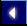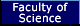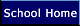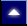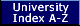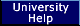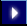Author: Simon Ambler (sja4@mcs.le.ac.uk).
Author: Steve Lakin (srl10@mcs.le.ac.uk).